# intercepting a ball.pptx

31 de May de 2023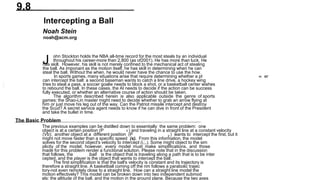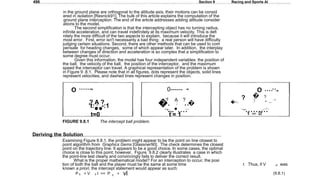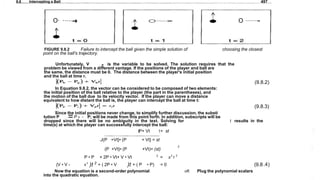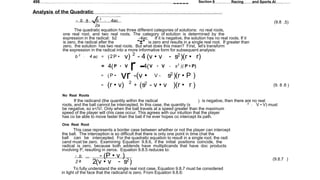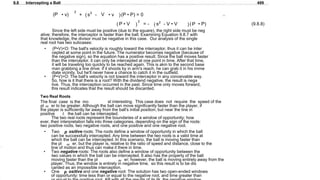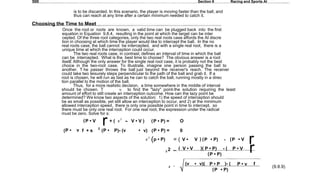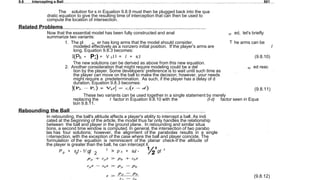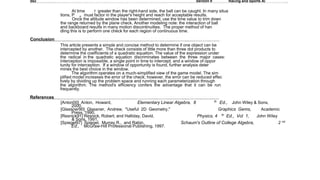1 de 8

### intercepting a ball.pptx

• 1. 9.8 Intercepting a Ball Noah Stein noah@acm.org J ohn Stockton holds the NBA all-time record for the most steals by an individual throughout his career-more than 2,800 (as of2001). He has more than luck. He has skill. However, his skill is not merely confined to the mechanical act of stealing the ball. As imponant as the motion itself, he has skill in determining when he can steal the ball. Without the when, he would never have the chance to use the how. In sports games, many situations arise that require determining whether a pl ay er can intercept the ball: a second baseman wants to catch a line drive, a hockey wing tries to steal a pass, a soccer goalie needs to block a shot, or a basketball center wishes to rebound the ball. In these cases, the AI needs to decide if the action can be success fully executed, or whether an alternative course of action should be taken. The algorithm described herein is also applicable outside the genre of sports games: the Shao-Lin master might need to decide whether to grab an arrow flying at him or just move his leg out of the way. Can the Patriot missile intercept and destroy the Scud? A secret service agent needs to know if he can dive in front of the President and take the bullet in time. The Basic Problem The previous examples can be distilled down to essentially the same problem: one object is at a certain position (P b ) and traveling in a straight line at a constant velocity (Vb); another object at a different position (P p ) wants to intercept the first, but it might not move faster than a specific speed (s). From this information, the model solves for the second object's velocity to intercept (i,;,). Some might object to the sim plicity of the model; however, every model must make simplifications, and those made for this problem render a functional solution. Please note that in the discussion that follows, the ball is the object that is traveling along a path that is to be inter cepted, and the player is the object that wants to intercept the ball. The first simplification is that the ball's velocity is constant and its trajectory is therefore a straight line. A basketball coming off the rim follows a parabolic trajec tory-not even remotely close to a straight line. How can a straight line model the motion effectively? This model can be broken down into two independent submod els: the altitude of the ball, and the motion in the ground plane. Because the two axes
• 2. 496 Section 9 Racing and Sports Al in the ground plane are orthogonal to the altitude axis, their motions can be consid ered in isolation [Resnick91]. The bulk of this article explains the computation of the ground plane interception. The end of the article addresses adding altitude consider ations to the model. The second simplification is that the intercepting object has no turning radius, infinite acceleration, and can travel indefinitely at its maximum velocity. This is defi nitely the more difficult of the two aspects to explain, because it will introduce the most error. First, error isn't necessarily a bad thing: a real person will have difficulty judging certain situations. Second, there are other methods that can be used to com pensate for heading changes, some of which appear later. In addition, the interplay between changes of direction and acceleration is so complex that a simplification to some degree must occur. Given this information, the model has four independent variables: the position of the ball, the velocity of the ball, the position of the interceptor, and the maximum speed the interceptor can travel. A graphical representation of the problem is shown in Figure 9 .8.1. Please note that in all figures, dots represent the objects, solid lines represent velocities, and dashed lines represent changes in position. O ······ ➔ ? A ? 't: : -:1 •• t=0 0------ ➔ ? A ? �. : ..� ·- ....•.... ·· t = 1 FIGURE 9.8.1 The intercept ball problem. O ······➔ � ? ? �--. • .,. -:1 ·--......... ····· Deriving the Solution Examining Figure 9.8.1, the problem might appear to be the point on line closest to point algorithm from Graphics Gems [Glassner90]. The check determines the closest point on the trajectory line. It appears to be a good choice. In some cases, the optimal choice is close to this point; however, Figure 9.8.2 clearly illustrates a case in which the point-line test clearly and convincingly fails to deliver the correct result. What is the proper mathematical model? For an interception to occur, the posi tion of both the ball and the player must be the same at some time t. Thus, if V P was known a priori, the intercept statement would appear as such: P b + V b t == P p + vl (9.8.1)
• 3. 9,8 Intercepting a Ball 497 O· ·····➔ O ······ ➔ FIGURE 9.8.2 Failure to intercept the ball given the simple solution of choosing the closest point on the ball's trajectory. Unfortunately, V P is the variable to be solved. The solution requires that the problem be viewed from a different vantage. If the positions of the player and ball are the same, the distance must be 0. The distance between the player's initial position and the ball at time r. (9.8.2) In Equation 9.8.2, the vector can be considered to be composed of two elements: the initial position of the ball relative to the player (the part in the parentheses), and the motion of the ball due to its velocity vector. If the player can move a distance equivalent to how distant the ball is, the player can intercept the ball at time t: (9.8.3) Since the initial positions never change, to simplify further discussion, the substi tution P =P b - P; will be made from this point forth. In addition, subscripts will be dropped since there will be no ambiguity in the text. Solving for t results in the time(s) at which the player can successfully intercept the ball: IP+ Vt != st .J(P +Vt}• (P + Vt} = st (P +Vt}• (P +Vt)= (st) 2 P • P + 2P • Vt+ V • Vt 2 = s2 t 2 (V • V - s 2 )t 2 + ( 2P • V )t + ( P • P) = 0 (9.8.4) Now the equation is a second-order polynomial oft. Plug the polynomial scalars into the quadratic equation.